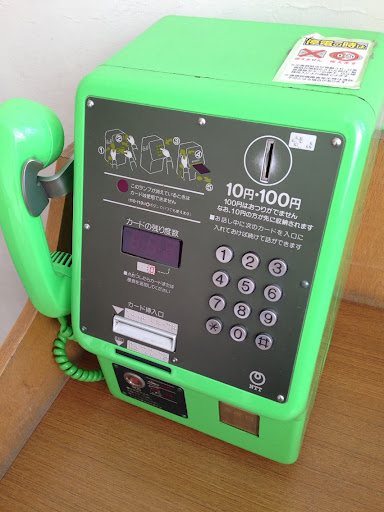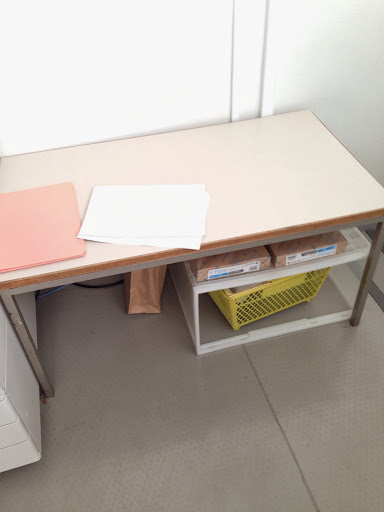# Revision blog post. (really.)|Math

###### *Sigh*

Hai. Hay. Horse eats hay.
This is a revision blog post. If you don’t like revision, turn 180° and leave your computer.

You okay?
Kay.

We are learning 2 concept in math. One is measurement. We learn things like how to  find the perimeter (the distance around the shape) or finding the area of a shape (height x length= area) like triangle, (h x l = a divided by 2) and other shapes. We also learn how to find a volume, (h x l x w = volume) and also we learn about time and measuring time. The other is probability. We learn about chances and in this concept we use a lot of decimals, fractions and percentage to show the probability. There are 5 words you use a lot in probability. One is impossible which means it will never happen. [It is impossible to get a wing and fly away right now] Another one is unlikely which means it is hard happen, [It is unlikely to win a lottery] and theres also even chance which means its fifty-fifty of happening [If you flips coin, there is a even chance of landing on tail or head] . The last two is Likely which means it has a high chance of happening [It is likely to be sunny today] and certain which means it will happen [It is certain that someone’s birthday is today].

I enjoyed a lot completing the probability activities. I got to learn more about probability and I actually got better at fractions, decimals and percentage from looking at my test scores. I found it easy to complete some probability activities and I got to help people out too.

I found it hard to find an area of some shapes like one of those that a rectangle and a triangle is connected together. Those were pretty annoying to solve and usually one of those careless mistake happens here. Its very time consuming to solve each question and I hope I can get better at it.

I think I want to get better at finding areas and volumes. I sometimes mistake and I hope I can fix that. I should start to double check the answer so I know its right. Also I should remember how to convert things (like mm to cm, cm to m) so I will memorize it perfect as possible before exam. For probability I should work on my reading skill. Yes my reading skill. Most of my mistakes I make it because I haven’t read the questions right so I should be careful during exam.

Finally its done.

Now I sleep.

# Areas and… Whats this post about again? | Math

Hellow.
I didn’t want to do this.
But I did it.
Pat on the back to me. (ﾉ｀△´)ﾉ ┫:･’∵:.┻┻:･’.:∵

Hai. Do you like math? No? I don’t care. were going to talk about measurements.

Our unit question is is: “How can we calculate using measurement?”. We can calculate the area of something by measuring. If you want to find the area of something, you measure the height and width and you multiply them.

We also use measurements in different environments. We obviously use rulers and other measuring instruments in school, but there are some other places we use those. Like people who work in architecture use measuring instruments all the time. They use rulers to measure the height, width, length, and they use scales to scale and see how heavy the materials are. Many people uses watches and clocks to see the time. In P.E we use stopwatch to record our time. Even in hospitals the shots have lines to measure and they also have thermometer to measure your temperature.

We have some questions about measurements too. What are the different units of measurements? How many are there in the whole world? How do you find the area of a circle? We know how to find the area of a square. But what about circles? How do find the area?Another question is: Why do we need to measure things? Why do we measure? What would the world be without measurements?

Now i’m going to show different types of units. First of is degrees. Degrees are used to measure temperatures or angles. °C (celsius) and °F (fahrenheit)  are used to measure temperatures. But ° are used to measure angles. For example: 90°, -15°C and 50°F.Another one is cm. This unit is very commonly used and they are used to measure width, height and length. Another common units are mm. Mm is equal to one tenth of a cm.Now i’m going to teach you how to find the area of a square. First you find the length and width of a square. Lets pretend the length and width of our square is 10cm. The height and the width is the same since its a square. Then you multiply them. 10 x 10 is 100 so the area for our square is 100cm^2.Now i’m going to show how to find the area of a rectangle. First we have to measure the width and length. Lets pretend the width of our rectangle is 10cm the length was 5cm. Then you multiply height and width together. 10 x 5 is 50 so the area for our rectangle is 50cm^2.Were going to try something trickier. I am going to show how to find the area of a triangle. First you have to find the height (base to the tip of the triangle) and the base. Lets say our base was 10cm long and the height was 11cm. We are going to multiply the height and the base together. 10 x 11 is 110. Now we are going to divide it into half. 250 divided by 2 is 125 so the answer is 110cm^2.Now i’m going to show how to find the area of a 3D rectangle. First you have to measure and find out the height, length and width. Then you multiply all 3 of them together. And BAHM, you have the area. Make sure to put ^3 in the end.

“were can we use measurement for good or evil?”
…What. How are we supposed to even classify measurements as good or evil?…. I have no idea.

If you want to find out how to get the area of a circle, go here because i’m too lazy to show you how.

…And now I pass out.

# 【Comparing Decimals】

Hai there,
How are you doing? Actually I don’t really care :3

Today we got in pairs and measured 3 objects outside and calculated how much it takes up spaces.

First off! Telephone-The length is 38.5cm and the width is 22.3cm.

38.5 x 22.3 = 858.55cm^2
Or in another way 0.085855m^2 (…what?)

Next is umbrella-The length is 60cm and the width is 10.2cm. (Source: Haruna Alice Doi)

60 x 10.2 = 612cm^2
Or in another way 61,200mm^2 (…..?)

And the last is a table!……. (Man it sounds really boring.)The length is 49.5cm and the width is 99.3cm. (Source: Haruna Alice Doi)

49.5 x 99.3 = 4915.35cm^2
Or in another way 491,535mm^2 (…I give up.)